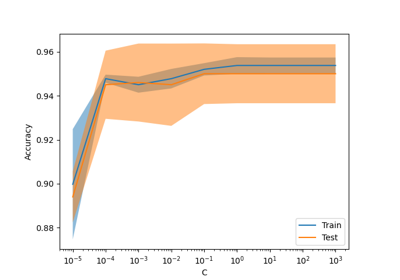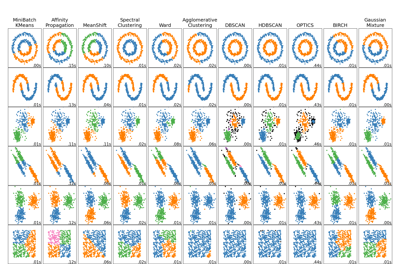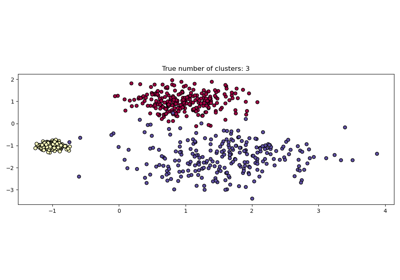# sklearn.cluster.HDBSCAN¶

class sklearn.cluster.HDBSCAN(min_cluster_size=5, min_samples=None, cluster_selection_epsilon=0.0, max_cluster_size=None, metric='euclidean', metric_params=None, alpha=1.0, algorithm='auto', leaf_size=40, n_jobs=None, cluster_selection_method='eom', allow_single_cluster=False, store_centers=None, copy=False)[source]

Cluster data using hierarchical density-based clustering.

HDBSCAN - Hierarchical Density-Based Spatial Clustering of Applications with Noise. Performs DBSCAN over varying epsilon values and integrates the result to find a clustering that gives the best stability over epsilon. This allows HDBSCAN to find clusters of varying densities (unlike DBSCAN), and be more robust to parameter selection. Read more in the User Guide.

For an example of how to use HDBSCAN, as well as a comparison to DBSCAN, please see the plotting demo.

New in version 1.3.

Parameters:
min_cluster_sizeint, default=5

The minimum number of samples in a group for that group to be considered a cluster; groupings smaller than this size will be left as noise.

min_samplesint, default=None

The number of samples in a neighborhood for a point to be considered as a core point. This includes the point itself. When None, defaults to min_cluster_size.

cluster_selection_epsilonfloat, default=0.0

A distance threshold. Clusters below this value will be merged. See  for more information.

max_cluster_sizeint, default=None

A limit to the size of clusters returned by the "eom" cluster selection algorithm. There is no limit when max_cluster_size=None. Has no effect if cluster_selection_method="leaf".

metricstr or callable, default=’euclidean’

The metric to use when calculating distance between instances in a feature array.

• If metric is a string or callable, it must be one of the options allowed by pairwise_distances for its metric parameter.

• If metric is “precomputed”, X is assumed to be a distance matrix and must be square.

metric_paramsdict, default=None

Arguments passed to the distance metric.

alphafloat, default=1.0

algorithm{“auto”, “brute”, “kdtree”, “balltree”}, default=”auto”

Exactly which algorithm to use for computing core distances; By default this is set to "auto" which attempts to use a KDTree tree if possible, otherwise it uses a BallTree tree. Both "KDTree" and "BallTree" algorithms use the NearestNeighbors estimator.

If the X passed during fit is sparse or metric is invalid for both KDTree and BallTree, then it resolves to use the "brute" algorithm.

leaf_sizeint, default=40

Leaf size for trees responsible for fast nearest neighbour queries when a KDTree or a BallTree are used as core-distance algorithms. A large dataset size and small leaf_size may induce excessive memory usage. If you are running out of memory consider increasing the leaf_size parameter. Ignored for algorithm="brute".

n_jobsint, default=None

Number of jobs to run in parallel to calculate distances. None means 1 unless in a joblib.parallel_backend context. -1 means using all processors. See Glossary for more details.

cluster_selection_method{“eom”, “leaf”}, default=”eom”

The method used to select clusters from the condensed tree. The standard approach for HDBSCAN* is to use an Excess of Mass ("eom") algorithm to find the most persistent clusters. Alternatively you can instead select the clusters at the leaves of the tree – this provides the most fine grained and homogeneous clusters.

allow_single_clusterbool, default=False

By default HDBSCAN* will not produce a single cluster, setting this to True will override this and allow single cluster results in the case that you feel this is a valid result for your dataset.

Which, if any, cluster centers to compute and store. The options are:

• None which does not compute nor store any centers.

• "centroid" which calculates the center by taking the weighted average of their positions. Note that the algorithm uses the euclidean metric and does not guarantee that the output will be an observed data point.

• "medoid" which calculates the center by taking the point in the fitted data which minimizes the distance to all other points in the cluster. This is slower than “centroid” since it requires computing additional pairwise distances between points of the same cluster but guarantees the output is an observed data point. The medoid is also well-defined for arbitrary metrics, and does not depend on a euclidean metric.

• "both" which computes and stores both forms of centers.

copybool, default=False

If copy=True then any time an in-place modifications would be made that would overwrite data passed to fit, a copy will first be made, guaranteeing that the original data will be unchanged. Currently, it only applies when metric="precomputed", when passing a dense array or a CSR sparse matrix and when algorithm="brute".

Attributes:
labels_ndarray of shape (n_samples,)

Cluster labels for each point in the dataset given to fit. Outliers are labeled as follows:

• Noisy samples are given the label -1.

• Samples with infinite elements (+/- np.inf) are given the label -2.

• Samples with missing data are given the label -3, even if they also have infinite elements.

probabilities_ndarray of shape (n_samples,)

The strength with which each sample is a member of its assigned cluster.

• Clustered samples have probabilities proportional to the degree that they persist as part of the cluster.

• Noisy samples have probability zero.

• Samples with infinite elements (+/- np.inf) have probability 0.

• Samples with missing data have probability np.nan.

n_features_in_int

Number of features seen during fit.

feature_names_in_ndarray of shape (n_features_in_,)

Names of features seen during fit. Defined only when X has feature names that are all strings.

centroids_ndarray of shape (n_clusters, n_features)

A collection containing the centroid of each cluster calculated under the standard euclidean metric. The centroids may fall “outside” their respective clusters if the clusters themselves are non-convex.

Note that n_clusters only counts non-outlier clusters. That is to say, the -1, -2, -3 labels for the outlier clusters are excluded.

medoids_ndarray of shape (n_clusters, n_features)

A collection containing the medoid of each cluster calculated under the whichever metric was passed to the metric parameter. The medoids are points in the original cluster which minimize the average distance to all other points in that cluster under the chosen metric. These can be thought of as the result of projecting the metric-based centroid back onto the cluster.

Note that n_clusters only counts non-outlier clusters. That is to say, the -1, -2, -3 labels for the outlier clusters are excluded.

DBSCAN

Density-Based Spatial Clustering of Applications with Noise.

OPTICS

Ordering Points To Identify the Clustering Structure.

Birch

Memory-efficient, online-learning algorithm.

References

Examples

>>> from sklearn.cluster import HDBSCAN
>>> hdb = HDBSCAN(min_cluster_size=20)
>>> hdb.fit(X)
HDBSCAN(min_cluster_size=20)
>>> hdb.labels_
array([ 2,  6, -1, ..., -1, -1, -1])


Methods

 dbscan_clustering(cut_distance[, ...]) Return clustering given by DBSCAN without border points. fit(X[, y]) Find clusters based on hierarchical density-based clustering. fit_predict(X[, y]) Cluster X and return the associated cluster labels. Get metadata routing of this object. get_params([deep]) Get parameters for this estimator. set_params(**params) Set the parameters of this estimator.
dbscan_clustering(cut_distance, min_cluster_size=5)[source]

Return clustering given by DBSCAN without border points.

Return clustering that would be equivalent to running DBSCAN* for a particular cut_distance (or epsilon) DBSCAN* can be thought of as DBSCAN without the border points. As such these results may differ slightly from cluster.DBSCAN due to the difference in implementation over the non-core points.

This can also be thought of as a flat clustering derived from constant height cut through the single linkage tree.

This represents the result of selecting a cut value for robust single linkage clustering. The min_cluster_size allows the flat clustering to declare noise points (and cluster smaller than min_cluster_size).

Parameters:
cut_distancefloat

The mutual reachability distance cut value to use to generate a flat clustering.

min_cluster_sizeint, default=5

Clusters smaller than this value with be called ‘noise’ and remain unclustered in the resulting flat clustering.

Returns:
labelsndarray of shape (n_samples,)

An array of cluster labels, one per datapoint. Outliers are labeled as follows:

• Noisy samples are given the label -1.

• Samples with infinite elements (+/- np.inf) are given the label -2.

• Samples with missing data are given the label -3, even if they also have infinite elements.

fit(X, y=None)[source]

Find clusters based on hierarchical density-based clustering.

Parameters:
X{array-like, sparse matrix} of shape (n_samples, n_features), or ndarray of shape (n_samples, n_samples)

A feature array, or array of distances between samples if metric='precomputed'.

yNone

Ignored.

Returns:
selfobject

Returns self.

fit_predict(X, y=None)[source]

Cluster X and return the associated cluster labels.

Parameters:
X{array-like, sparse matrix} of shape (n_samples, n_features), or ndarray of shape (n_samples, n_samples)

A feature array, or array of distances between samples if metric='precomputed'.

yNone

Ignored.

Returns:
yndarray of shape (n_samples,)

Cluster labels.

Get metadata routing of this object.

Please check User Guide on how the routing mechanism works.

Returns:

A MetadataRequest encapsulating routing information.

get_params(deep=True)[source]

Get parameters for this estimator.

Parameters:
deepbool, default=True

If True, will return the parameters for this estimator and contained subobjects that are estimators.

Returns:
paramsdict

Parameter names mapped to their values.

set_params(**params)[source]

Set the parameters of this estimator.

The method works on simple estimators as well as on nested objects (such as Pipeline). The latter have parameters of the form <component>__<parameter> so that it’s possible to update each component of a nested object.

Parameters:
**paramsdict

Estimator parameters.

Returns:
selfestimator instance

Estimator instance.

## Examples using sklearn.cluster.HDBSCAN¶Release Highlights for scikit-learn 1.3

Release Highlights for scikit-learn 1.3Comparing different clustering algorithms on toy datasets

Comparing different clustering algorithms on toy datasetsDemo of HDBSCAN clustering algorithm

Demo of HDBSCAN clustering algorithm